# A Hybrid Model Integrating Singular Spectrum Analysis and Backpropagation Neural Network for Stock Price Forecasting

A Hybrid Model Integrating Singular Spectrum Analysis and Backpropagation Neural Network for Stock Price Forecasting
Department of Operations Research and Decision Support, Faculty of Computers and Information, Cairo University, Orman 12613, Giza, Egypt

Department of Petroleum Engineering, Universiti Teknologi PETRONAS (UTP), Seri Iskandar 32610, Perak Darul Ridzuan, Malaysia

Corresponding Author Email:
a.fathi@fci-cu.edu.eg
Page:
483-488
|
DOI:
https://doi.org/10.18280/ria.350606
29 September 2021
|
Accepted:
3 December 2021
|
Published:
28 December 2021
| Citation

OPEN ACCESS

Abstract:

The primary purpose of trading in stock markets is to profit from buying and selling listed stocks. However, numerous factors can influence the stock prices, such as the company's present financial situation, news, rumor, macroeconomics, psychological, economic, political, and geopolitical factors. Consequently, tremendous challenges already exist in predicting noisy stock prices. This paper proposes a hybrid model integrating the singular spectrum analysis (SSA) and the backpropagation neural network (BPNN) to forecast daily closing prices in stock markets. The model first decomposes the stock prices into several components using the SSA. Then, the extracted components are utilized for training BPNNs to forecast future prices. Compared with the BPNN, the hybrid SSA-BPNN model demonstrates a better predictive performance, indicating the SSA's ability to extract hidden information and reduce the noise effect of the original time series.

Keywords:

stock market, stock price prediction, singular spectrum analysis, neural network, hybrid model

1. Introduction

The stock market is a public entity that provides financial activities to buy, sell, and issue shares of publicly traded companies . A stock is a financial instrument that denotes ownership in a company and grants stockholders a portion of the company's assets and earnings. The company's stock is divided into shares at the initial public offering. Consequently, a share represents the smallest ownership unit in a particular company . The initial price of a company's stock is determined by the company's value, revenues, and financial situation at the initial public offering. Afterward, its value fluctuates according to the stock's supply and demand. When a trader buys any number of shares of a company's stock, the expression "entered the market" is frequently used. If the price of the purchased stock moves, the actual worth of the investment changes. When the stock price increases, the trader will be in floating profit. In contrast, when the stock's value declines, the trader will be in floating loss. The final profit or loss is not realized until the trade is closed. Therefore, a trader must understand how stocks should be valued to purchase or sell stocks if their current value is less or greater than the fair value .

The stock market presents opportunities for individuals to invest in companies. The primary purpose of trading in stock markets is to profit from buying and selling listed stocks; however, it is considered a high-risk, high-yield investment . In the last decade, soft computing models, e.g., artificial neural networks (ANN) and support vector machine (SVM) models, have shown to be effective tools for financial market prediction . According to the works [5, 6], ANN models are the most commonly used to forecast various financial markets. ANN is a machine learning technique that simulates the human brain's structure and operation . ANNs can precisely predict and distinguish nonlinear dataset patterns without prior knowledge, leading to their broad acceptability and adjustability [7-9]. Moreover, ANNs possess learning, generalization, and parallel processing features that efficiently resolve complex problems . Consequently, ANNs are suitable for modeling time series characterized by significant fluctuations and discontinuities . Unfortunately, most systems arising in practice, such as stock prices, are time-varying [12, 13] and contain much noise [14, 15], which reduces the ANNs' prediction efficiency. Consequently, data preprocessing techniques like singular spectrum analysis (SSA) are required to reduce the noise and extract interesting underlying information from the original time series [16, 17].

The SSA utilizes the singular value decomposition (SVD) to decompose time series and acquire a set of singular values that hold information about the original data [18-20]. The decomposed components are distinguished as a trend, periodic, or noise [21, 22]. Hassani et al.  utilized the SSA to predict the British pound versus United states dollar daily exchange rate. The author concluded that the SSA model outperformed random walk models in forecasting exchange rate series. Menezes et al. explored the market linkages among the G7 countries via analyzing the stock market data based on SSA . Wen et al.  introduced a hybrid model for predicting stock prices using SSA and SVM. The authors compared the predictive performance of the SSA-SVM to the single SVM model and discovered that the hybrid model outperforms the SVM prediction. Abdollahzade et al.  integrated the neuro-fuzzy models with SSA optimized by particle swarm to predict nonstationary chaotic time series. The proposed model was found to perform better in predicting nonlinear time series compared to various other methods. Lahmiri suggested a hybrid technique to predict intraday stock prices that combines SSA and SVR with particle swarm optimization (PSO) . The suggested model's performance was validated in six intraday stock price series and demonstrated significant potential for analyzing and forecasting noisy time series. Xiao et al. combined the SSA and SVM to analyze and predict stock prices and applied them to the stock price of the Shanghai Stock Exchange (SSE) Composite Index . Sulandari et al. presented a methodology for time series forecasting based on SSA and ANN and concluded that hybrid models outperformed single models .

In this study, a hybrid model integrating SSA and BPNN is developed to predict daily closing prices in stock markets. The model utilizes the SSA to decompose the stock prices to extract hidden information from the original time series and reduce the noise effect. For each decomposed component, a BPNN is constructed and trained to forecast one day ahead. The forecasted components are then aggregated to produce the final output. Unlike previous studies, the stock prices are first split into training and testing datasets; hence the testing set is hidden during the decomposition process. The proposed SSA-BPNN was validated using experimental data of the largest twenty market capital stocks listed in the Nasdaq stock exchange.

2. Research Methods

2.1 Singular spectrum analysis (SSA)

The SSA has various applications, such as denoising signals, extracting the underlying trend, and forecasting applications . The SSA approach comprises two interrelated stages, i.e., decomposition and reconstruction, and each comprises two steps . The first stage involves decomposing the time series into simple and meaningful signals through embedding succeeded by SVD . The second stage includes reconstructing the series for forecasting purposes via grouping and diagonal averaging . This section summarizes the different stages and parameters selection criteria of the SSA.

2.1.1 Decomposition

The decomposition process is divided into two steps, which are embedding and SVD. Embedding is a preliminary step in analyzing time series that converts the original one-dimensional series to a lagged multi-dimensional trajectory matrix . For example, let St = [S1, S2, …, SN]T be a time-series of length N, then the mapped trajectory matrix, with dimensions L×K, is defined as [28, 29]:

$X=\left[X_{1}, X_{2}, \ldots, X_{K}\right]=\left(\begin{array}{cccc}S_{1} & S_{2} & \cdots & S_{K} \\ S_{2} & S_{3} & \cdots & S_{K+1} \\ \vdots & \vdots & \ddots & \vdots \\ S_{L} & S_{L+1} & \cdots & S_{N}\end{array}\right)$                   (1)

where, L is the window length representing the embedding dimension (2 ≤ L ≤ N), and K = N-L+1. The trajectory matrix X is a Hankel matrix since the elements along its antidiagonals are identical.

Following the embedding process, the SVD is utilized to factorize the trajectory matrix into biorthogonal elementary matrices . This process is expressed as :

$X=\sum_{r=1}^{R} X_{r}=U \Sigma V^{T}=\sum_{r=1}^{R} \sigma_{r} u_{r} v_{r}^{T}$             (2)

where, $X_{r}$ is the rth elementary matrix, U and V form an orthonormal system, Σ is a diagonal matrix whose diagonal elements, $\sigma_{r}=\sqrt{\lambda_{r}}$, are the singular values of the lag-covariance matrix XXT, $u_{r}$ denotes the eigenvector corresponding to the eigenvalue $\lambda_{r}, v_{r}$ represents the rth principal component, and R is the rank of X. The set $\left(\sigma_{r}, u_{r}, v_{r}\right)$ is known as the rth eigentriple of the trajectory matrix.

2.1.2 Reconstruction

Reconstruction is the second stage of the SSA, in which the time-series is projected onto data-adaptive eigenvectors that reduce the dimensionality of the dataset via representing it in an optimal subspace . The reconstruction process is divided into two steps, which are grouping and diagonal averaging. Grouping involves categorizing the elementary matrices, Xi, according to their eigentriples into groups. Then the matrices inside each group are summed up [26, 27]. Let g = {i1, i2, …, ir} be a group of r selected eigentriples; hence, the matrix Xg for group g is expressed as:

$X_{g}=X_{i_{1}}+X_{i_{2}}+\cdots+X_{i_{r}}$        (3)

The increment of singular entropy due to eigentriple i is represented by Eq. (4). The information within the time series is considered extracted when ΔE reaches an asymptotic value, and the following components are therefore caused by noise.

$\Delta E=-\left(\frac{\lambda_{i}}{\sum_{r=1}^{p} \lambda_{r}}\right) \log \left(\frac{\lambda_{i}}{\sum_{r=1}^{p} \lambda_{r}}\right)$           (4)

The second step in the reconstruction process is the diagonal averaging along the N antidiagonal of the matrix Xg. This process transfers the matrix into a time series that will be a component of the original series St. The reconstructed time series will have a length N. Finally, the correlation coefficient between different components is utilized to determine their linear dependence. The correlation coefficient, ρ, in terms of the covariance of two components $X_{g_{1}}$ and $X_{g_{2}}$ is:

$\rho=\frac{\operatorname{cov}\left(X_{g_{1}}, X_{g_{2}}\right)}{\sigma_{1} \sigma_{2}}$         (5)

where, σ is the component's standard deviations. In this work, components with ρ ≥ 0.4 are aggregated.

2.2 Backpropagation neural networks (BPNN)

The artificial neural network comprises simple connected processors referred to as neurons, resembling the human brain's neurons. The neurons are joined by weighted connections that transfer signals between them. A neuron receives several input signals via its connections yet only produces one output signal. Then, the neuron's output signal is transferred via its outgoing connection into multiple branches that send identical signals. The outgoing branches terminate at other neurons' incoming connections . The neuron's output is determined via an activation function that compares its calculated input to a predetermined threshold value.

There have been several investigations on activation functions in the literature, only a few have demonstrated their usefulness. For example, step and sign activation functions are frequently utilized in decision-making neurons to perform classification and pattern recognition tasks. On the other hand, the sigmoid function converts an input number between plus and minus infinity to a sensible value between zero and one. The linear activation function produces an output proportional to the neuron's weighted input. Generally, neurons with linear activation functions are frequently utilized to approximate linear functions .

A BPNN is a multilayer network with one or more hidden layers trained using a backpropagation learning algorithm (Figure 1). The network is typically composed of an input layer of source neurons, at least a hidden layer of computational neurons, and an output layer of computational neurons. The input signals are transmitted forward through successive layers. Each layer in a multilayer neural network serves a distinct purpose. The input layer receives signals from the external environment and distributes them to all neurons in the hidden layer. Indeed, because the input layer rarely contains computing neurons, it does not process input patterns. The hidden layer's neurons recognize the characteristics concealed in the input signals. Then, the output layer receives output signals from the hidden layer and establishes the output pattern for the entire network. The backpropagation learning algorithm is widely used for ANNs learning and is divided into two phases . First, the network input layer is supplied with training data. After that, the network propagates the input data across each layer till the output layer generates the network's output. If this output does not match the required training data output, the error is measured and sent backward across the network from the output layer to the input layer. Weights are tuned in real-time as the error propagates.

## 1.png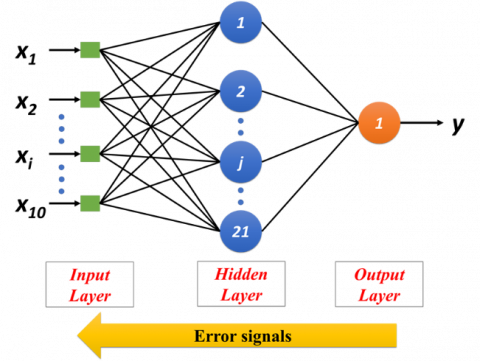Figure 1. A three-layers BPNN

In this work, the daily closing prices are predicted by considering the ten preceding prices; hence there are ten input neurons in the input layer. According to Huang and Wang (2018), the number of the hidden neurons can be set approximately to be 2×n+1, where n is the number of the input neurons, and 1 denotes an output neuron . Correspondingly, we adopt a 10×21×1 structure of the BPNNs, as shown in Figure 1.

2.3 The hybrid SSA-BPNN model

This paper integrates the SSA and the BPNN to build a hybrid forecasting model, i.e., the SSA-BPNN model. First, the model utilizes the SSA to decompose the stock prices into several components. Then, the decomposed components are fed to the BPNNs to forecast one step of future daily prices. Finally, the forecasted outputs from the BPNNs are aggregated to produce the final prediction. Figure 2 shows a flow chart of the hybrid SSA-BPNN model, and the procedures are detailed as:

1. Split the daily closing prices into a training dataset (70%) and a testing dataset (30%).
2. Decompose the training dataset by the SSA using window length, L = 14.
3. Calculate the increment of singular entropy, $\Delta E$, of various singular values.
4. Group elementary matrices when $\Delta E$ reaches an asymptotic value.
5. Reconstruct time series components.
6. Aggregate linearly dependent components (ρ ≥ 0.4).
7. Normalize the reconstructed market components.
8. Build and train three BPNNs (10×21×1) to predict each market component.
9. Forecast the future price of each point in the testing dataset using the following steps:
1. Decompose its prior prices as shown in steps 2 - 7.
2. Forecast one step of each component.
3. Denormalize and aggregate the forecasted components.
4. Repeat steps (a) to (d) until all the testing points are predicted.

## 2.png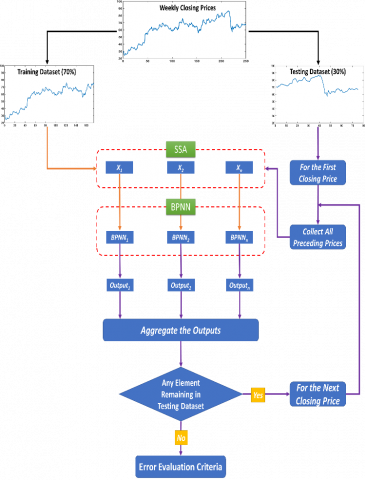Figure 2. Flow chart of SSA-BPNN model

3. Results and Discussion

The data of the largest twenty market capital stocks listed in the Nasdaq stock exchange are utilized to validate the SSA-BPNN model. The data comprises the daily closing prices from January 2nd, 2015, to December 31st, 2019. There are 1007 trading days for each dataset. For illustration, the SSA decomposition process of the AAPL stock prices is detailed. The first 70% of the stock prices are chosen as the training set, while the remaining 30% comprise the testing dataset. The training series is decomposed into 14 elementary matrices, and the increment of singular entropy is calculated for each matrix. As shown in Figure 3, the increment of singular entropy saturates at the eighth order, and the difference in entropy between two consecutive orders is lower than 10-5. Therefore, elementary matrices from 8 to 14 are combined to form the noise term.

The next step is reconstructing the different matrices and calculating the correlation coefficient, as shown in Table 1. The correlation coefficient between reconstructed components one and two is -0.0013, indicating that they are separable. Similarly, components 7 and 8 are separable. In contrast, components 2 through 7 have higher correlation coefficients and are combined in one component. Figure 4 displays the final reconstructed three components, i.e., RC1, RC2, and RC3, of the AAPL. RC1 represents the market trend. The medium oscillatory component RC2 is considered the market fluctuation, while the high oscillatory component RC3 indicates market noise.

Each reconstructed component is utilized for training a BPNN. Then the trained BPNNs are used to predict the various components. Finally, the forecasted components are aggregated to produce the forecasted prices. The mean average error (MAE), root mean square error (RMSE), and the mean absolute percentage error (MAPE) are employed to evaluate the prediction performances, as shown in Table 2.

## 3.png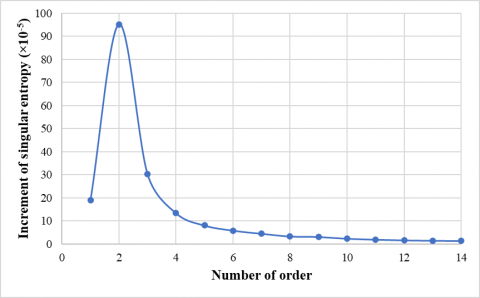Figure 3. Increment of the singular entropy

## 4.png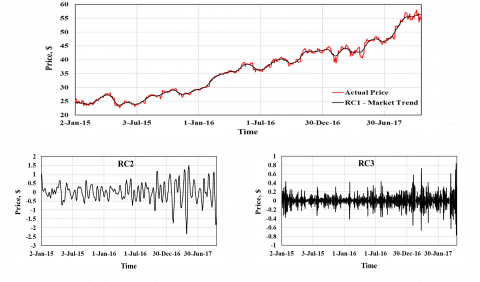Figure 4. AAPL stock prices and decomposed components

Table 1. Correlation coefficients between the eight reconstructed components

 Reconstructed Matrix 1 2 3 4 5 6 7 8 1 1 -0.0013 -0.0112 0.0185 -0.0066 0.0026 -0.0076 0.0007 2 -0.0013 1 0.6528 0.0479 0.0466 0.0092 0.0277 0.0052 3 -0.0112 0.6528 1 0.5229 0.1686 0.0553 0.0286 0.0139 4 0.0185 0.0479 0.5229 1 0.588 0.1234 0.0683 0.0261 5 -0.0066 0.0466 0.1686 0.588 1 0.5473 0.1646 0.0398 6 0.0026 0.0092 0.0553 0.1234 0.5473 1 0.6298 0.0807 7 -0.0076 0.0277 0.0286 0.0683 0.1646 0.6298 1 0.2347 8 0.0007 0.0052 0.0139 0.0261 0.0398 0.0807 0.2347 1

Table 2. Forecasting performance of the proposed SSA-BPNN and BPNN for individual stocks

 Stock MAE RMSE MAPE BPNN SSA-BPNN BPNN SSA-BPNN BPNN SSA-BPNN AAPL 2.10 0.94 3.63 1.27 3.63 1.82 MSFT 10.64 3.25 13.98 4.25 7.70 2.44 GOOG 26.54 17.84 38.35 23.82 2.29 1.56 GOOGL 16.40 16.13 23.03 21.65 1.43 1.40 AMZN 36.34 28.45 58.23 40.28 2.13 1.67 FB 3.01 2.79 4.05 3.70 1.74 1.66 TSLA 1.64 1.56 2.37 2.17 2.91 2.76 NVDA 1.21 1.20 1.98 1.72 2.91 2.80 ASML 5.54 4.78 7.45 6.50 2.61 2.19 ADI 4.96 1.75 7.47 2.26 4.65 1.71 PYPL 3.87 3.19 5.24 4.00 3.71 3.04 ADBE 6.79 4.20 9.07 5.60 2.54 1.57 CMCSA 0.87 0.46 1.20 0.60 2.04 1.13 NFLX 7.68 7.45 10.25 9.78 2.47 2.36 CSCO 0.78 0.72 1.05 0.97 1.57 1.45 INTC 0.98 0.85 1.29 1.19 1.95 1.70 PEP 11.89 3.55 15.70 4.58 8.94 2.70 COST 31.48 9.80 44.51 12.97 11.06 3.61 AVGO 6.64 5.59 8.39 7.11 2.36 2.05 TXN 2.71 1.70 3.85 2.32 2.34 1.55

Figure 5 displays a box plot for MAPE of BPNN and SSA-BPNN models. The SSA-BPNN model has the lowest median and mean MAPE for the twenty stocks involved in the analysis. Also, the MAPE for 75% of the stocks is lower than 2.8%. Moreover, the hybrid model does not have any outliers.

## 5.png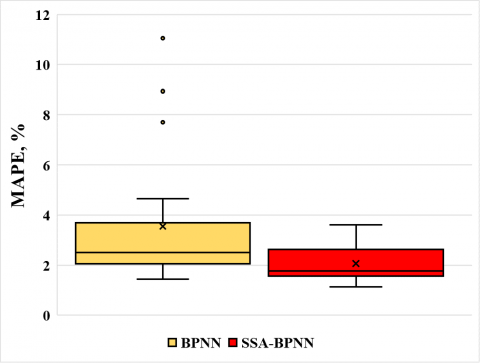Figure 5. Box plot of MAPE for BPNN and SSA-BPNN models

From the previous analysis, it is evident that the proposed hybrid SSA-BPNN outperforms the single BPNN in terms of MAE, RMSE, and MAPE when predicting daily closing prices. The improved performance of the hybrid SSA-BPNN model is attributed to the SSA's ability to extract hidden information and reduce the noise effect in the original time series.

4. Conclusion

This paper proposed a hybrid forecasting model based on SSA and BPNN to predict daily closing prices in stock markets. The model employs the SSA to decompose the stock prices to reduce the noise and extract hidden information from the original price series. Also, BPNNs are built and trained to predict one day ahead of the different decomposed components. The BPNNs' outputs are summed up to produce the final forecasted prices.

The proposed model has been proven as an effective tool for predicting stock prices via conducting an empirical study with twenty stocks listed in the Nasdaq stock exchange. The research results indicated that the proposed SSA-BPNN model obtained the lowest MAE, MAPE, and RMSE for all stocks involved in the analysis. Furthermore, the model's superior forecasting ability is associated with its strength in capturing hidden information in financial time series, such as trends and volatility. Therefore, the presented approach is a promising tool for predicting daily closing prices.

References

 Göçken, M., Özçalıcı, M., Boru, A., Dosdoğru, A.T. (2016). Integrating metaheuristics and artificial neural networks for improved stock price prediction. Expert Systems with Applications, 44: 320-331. https://doi.org/10.1016/j.eswa.2015.09.029

 Abirami, R., Vijaya, M.S. (2011). Stock price prediction using support vector regression. In International Conference on Computing and Communication Systems, pp. 588-597. https://doi.org/10.1007/978-3-642-29219-4_67

 Wang, Y., Xing, H. (2011). Time interval analysis on price prediction in stock market based on general regression neural networks. In International Conference on Electronic Commerce, Web Application, and Communication, pp. 160-166. http://dx.doi.org/10.1007/978-3-642-20370-1_27

 Kara, Y., Boyacioglu, M.A., Baykan, Ö.K. (2011). Predicting direction of stock price index movement using artificial neural networks and support vector machines: The sample of the Istanbul Stock Exchange. Expert systems with Applications, 38(5): 5311-5319. https://doi.org/10.1016/j.eswa.2010.10.027.

 Lin, X., Yang, Z., Song, Y. (2009). Short-term stock price prediction based on echo state networks. Expert systems with applications, 36(3): 7313-7317. https://doi.org/10.1016/j.eswa.2008.09.049

 Senapati, M.R., Das, S., Mishra, S. (2018). A novel model for stock price prediction using hybrid neural network. Journal of the Institution of Engineers (India): Series B, 99(6): 555-563. http://dx.doi.org/10.1007/s40031-018-0343-7

 Jothimani, D., Shankar, R., Yadav, S.S. (2015). A hybrid EMD-ANN model for stock price prediction. In International Conference on Swarm, Evolutionary, and Memetic Computing, pp. 60-70. http://dx.doi.org/10.1007/978-3-319-48959-9_6

 Büyükşahin, Ü.Ç., Ertekin, Ş. (2019). Improving forecasting accuracy of time series data using a new ARIMA-ANN hybrid method and empirical mode decomposition. Neurocomputing, 361: 151-163. http://dx.doi.org/10.1016/j.neucom.2019.05.099

 Safari, A., Davallou, M. (2018). Oil price forecasting using a hybrid model. Energy, 148: 49-58. http://dx.doi.org/10.1016/j.energy.2018.01.007

 Wang, J.Z., Wang, J.J., Zhang, Z.G., Guo, S.P. (2011). Forecasting stock indices with back propagation neural network. Expert Systems with Applications, 38(11): 14346-14355. https://doi.org/10.1016/j.eswa.2011.04.222

 Ruiz, L.G.B., Cuéllar, M.P., Calvo-Flores, M.D., Jiménez, M.D.C.P. (2016). An application of non-linear autoregressive neural networks to predict energy consumption in public buildings. Energies, 9(9): 684. https://doi.org/10.3390/en9090684

 Abdelaziz, T.H. (2018). Stabilization of linear time-varying systems using proportional-derivative state feedback. Transactions of the Institute of Measurement and Control, 40(7): 2100-2115. https://doi.org/10.1177/0142331217697787

 Abdelaziz, T.H. (2016). Eigenstructure assignment by displacement–acceleration feedback for second-order systems. Journal of Dynamic Systems, Measurement, and Control, 138(6). https://doi.org/10.1115/1.4032877

 Wei, L.Y. (2016). A hybrid ANFIS model based on empirical mode decomposition for stock time series forecasting. Applied Soft Computing, 42: 368-376. https://doi.org/10.1016/j.asoc.2016.01.027

 Lu, C.J., Chiu, C.C., Yang, J.L. (2009). Integrating nonlinear independent component analysis and neural network in stock price prediction. In International Conference on Industrial, Engineering and Other Applications of Applied Intelligent Systems, pp. 614-623. https://doi.org/10.1007/978-3-642-02568-6_62

 Lu, C.J. (2013). Hybridizing nonlinear independent component analysis and support vector regression with particle swarm optimization for stock index forecasting. Neural Computing and Applications, 23(7): 2417-2427. https://doi.org/10.1007/s00521-012-1198-5

 Kao, L.J., Chiu, C.C., Lu, C.J., Yang, J.L. (2013). Integration of nonlinear independent component analysis and support vector regression for stock price forecasting. Neurocomputing, 99: 534-542. https://doi.org/10.1016/j.neucom.2012.06.037

 Lahmiri, S. (2018). Minute-ahead stock price forecasting based on singular spectrum analysis and support vector regression. Applied Mathematics and Computation, 320: 444-451. https://doi.org/10.1016/j.amc.2017.09.049

 Wen, F.H., Xiao, J.H., He, Z. F., Gong, X. (2014). Stock price prediction based on SSA and SVM. Procedia Computer Science, 31: 625-631. https://doi.org/10.1016/j.procs.2014.05.309

 Trendafilova, I. (2021). Singular spectrum analysis for the investigation of structural vibrations. Engineering Structures, 242: 112531. https://doi.org/10.1016/j.engstruct.2021.112531

 Hassani, H., Soofi, A.S., Zhigljavsky, A.A. (2010). Predicting daily exchange rate with singular spectrum analysis. Nonlinear Analysis: Real World Applications, 11(3): 2023-2034. https://doi.org/10.1016/j.nonrwa.2009.05.008

 Abdollahzade, M., Miranian, A., Hassani, H., Iranmanesh, H. (2015). A new hybrid enhanced local linear neuro-fuzzy model based on the optimized singular spectrum analysis and its application for nonlinear and chaotic time series forecasting. Information Sciences, 295: 107-125. https://doi.org/10.1016/j.ins.2014.09.002

 Menezes, R., Dionísio, A., Hassani, H. (2012). On the globalization of stock markets: An application of vector error correction model, mutual information and singular spectrum analysis to the G7 countries. The Quarterly Review of Economics and Finance, 52(4): 369-384. https://doi.org/10.1016/j.qref.2012.10.002

 Xiao, J., Zhu, X., Huang, C., Yang, X., Wen, F., Zhong, M. (2019). A new approach for stock price analysis and prediction based on SSA and SVM. International Journal of Information Technology & Decision Making, 18(1): 287-310. https://doi.org/10.1142/S021962201841002X

 Sulandari, W., Subanar, S., Lee, M.H., Rodrigues, P.C. (2020). Time series forecasting using singular spectrum analysis, fuzzy systems and neural networks. MethodsX, 7: 101015. https://doi.org/10.1016/j.mex.2020.101015

 Lin, Y., Ling, B.W.K., Xu, N., Lam, R.W.K., Ho, C.Y.F. (2020). Effectiveness analysis of bio-electronic stimulation therapy to Parkinson’s diseases via joint singular spectrum analysis and discrete fourier transform approach. Biomedical Signal Processing and Control, 62: 102131. https://doi.org/10.1016/j.bspc.2020.102131

 Hassani, H. (2007). Singular spectrum analysis: Methodology and comparison. Journal of Data Sciences, 5: 239-257. https://doi.org/10.6339/JDS.2007.05(2).396

 Rocco S.C.M. (2013). Singular spectrum analysis and forecasting of failure time series. Reliability Engineering and System Safety, 114: 126-136. https://doi.org/10.1016/j.ress.2013.01.007

 De Carvalho, M., Rua, A. (2017). Real-time nowcasting the US output gap: Singular spectrum analysis at work. International Journal of Forecasting, 33(1): 185-198. https://doi.org/10.1016/j.ijforecast.2015.09.004

 Leles, M.C., Sansão, J.P.H., Mozelli, L.A., Guimarães, H.N. (2018). Improving reconstruction of time-series based in Singular Spectrum Analysis: A segmentation approach. Digital Signal Processing, 77: 63-76. https://doi.org/10.1016/j.dsp.2017.10.025

 Groth, A., Ghil, M. (2015). Monte Carlo singular spectrum analysis (SSA) revisited: Detecting oscillator clusters in multivariate datasets. Journal of Climate, 28(19): 7873-7893. https://doi.org/10.1175/JCLI-D-15-0100.1

 Negnevitsky, M. (2005). Artificial Intelligence: A Guide to Intelligent Systems. Pearson education.

 Huang, L., Wang, J. (2018). Forecasting energy fluctuation model by wavelet decomposition and stochastic recurrent wavelet neural network. Neurocomputing, 309, 70-82. https://doi.org/10.1016/j.neucom.2018.04.071